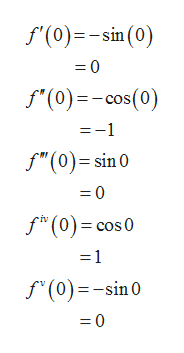Question
1 views

Derive the Taylor series for cos x centered at a=0.

check_circle

Step 1

The Taylor series for the function f(x) centered at a is given by,

Step 2

Let f(x) = cos x. Then compute the derivatives as follows.

Step 3

Substitute x = 0 in the derivatives and ...help_outlineImage Transcriptionclosef'(0)sin (0) =0 f(0)cos(0) =-1 f"(0) sin 0 0 f (0) cos 0 -1 f(0)sin0 0 fullscreen

### Want to see the full answer?

See Solution

#### Want to see this answer and more?

Solutions are written by subject experts who are available 24/7. Questions are typically answered within 1 hour.*

See Solution
*Response times may vary by subject and question.
Tagged in

### Other